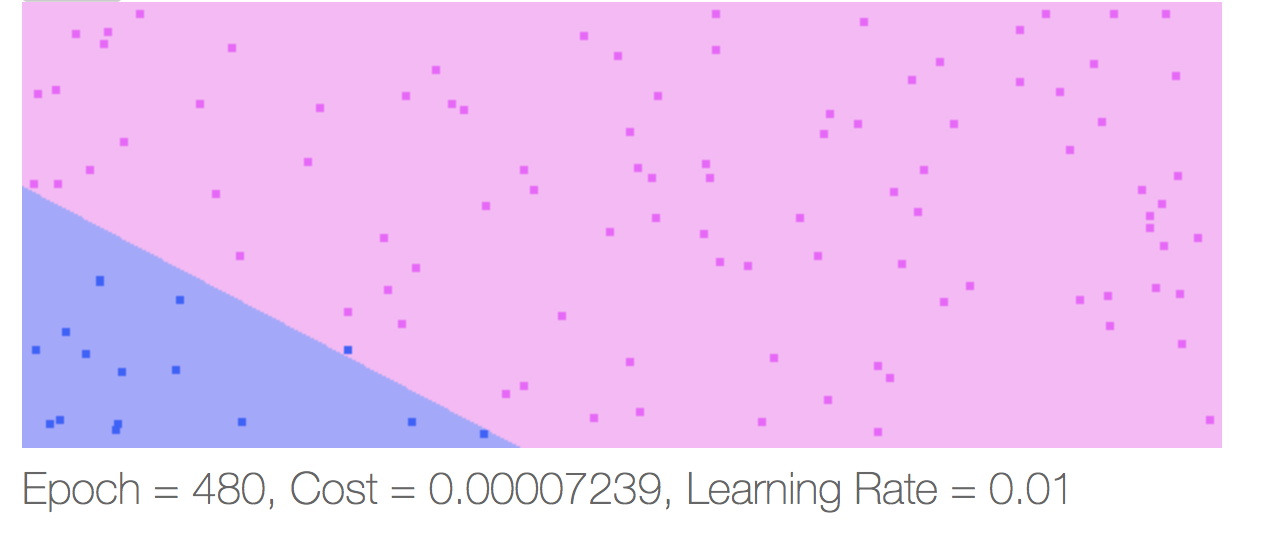# Rodrigo Silveira

## Machine Learning - Linear ClassifierThis is an old demo I made in 2013.

An interactive demo showing linear regression fitting a very separable dataset. This work was very early practice as I was learning the foundations of supervised learning.

As mentioned in other posts… last night (November 4, 2020) I was showing my 7 year old daughter what Twitter is. I decided to show her some of the weird stuff I’d posted over the years. One of the posts was a link to an old demo I had posted on an old version of this blog. I clicked on the link, only to realize I’d removed all my old blog posts not related to my current pursuit of AI and Machine Learning. My daughter told me that I “should never delete stuff, because then people would never know what I had to say or show them.”

That 7 year old wisdom was enough motivation for me to dig through my Github account and find the code for that and other demos, which is what you’ll find below.

# The original content

After building a linear regression model/trainer, the next natural step was to extend the JavaScript library to perform linear classification. Although making the library perform multi-class classification, this particular implementation only classifies between two classes.

## Demo: Learning to classify two classes

This toy application is given random points throughout a two dimensional grid. A random line is selected to separate the points. Any points above the line are assigned a color, and points below the line are assigned a different color. The purpose of the algorithm is to learn which points belong to each class. After each learning iteration, the app classifies every point in the grid. The background color is pointed based on the class the algorithm classifies.

## Implementation details

With this being my first attempt to implement a logistic regression model in JavaScript, I was surprised at how simple it really was to do. The training code consists of a few lines of code:

SigmoidClassifier.prototype.train = function (samples, labels, config = { learningRate: 0.05, epochs: 10 }) {
const learningRate = config.learningRate;
const maxEpochs = config.epochs;
const M = samples.length;
let epoch = 0;

const lr = learningRate / M;
const costFrac = -1 / M;

while (epoch++ < maxEpochs) {
const scores = samples.map(sample => this.score(sample));
const errors = scores.map((score, i) => score - labels[i]);
this.params = this.params.map((param, col) => {
return param - lr * errors.reduce((acc, error, row) => (acc + error * samples[row][col]), 0);
});
}
};


In summary, the update for each weight is performed by simultaneously updating all weights as follows:

$\theta_{j} := \theta_{j} - \alpha \sum^m_{i=1}(h_\theta(x^{(i)}) - y^{(i)})x^{(i)}_j$

That is:

• $$\alpha$$ is the learning rate
• $$h_\theta(x^{(i)})$$ is the prediction for the ith sample
• $$y^{(i)}$$ is the actual label for the ith sample
• $$\theta_{j}$$ is the jth weight in the model

For completion, the score function is a simple sigmoid activator implemented as

SigmoidClassifier.prototype.score = function (inputs) {
const sum = inputs.reduce((acc, input, i) => {
return acc + input * this.params[i];
}, 0);

return 1 / (1 + Math.exp(-sum));
};


My next goal is now to write a simple neural network so I can perform the same classification concept, but with a non-linear data set.

## Lessons learned

The main takeaway I got from this exercise was that the size of the input data is key. Concretely, I had features that ranged between 0-600 (the width in pixels of my grid). The problem this caused is that I’d get Infinity when computing the score of a few points at the upper end of that range. A simple solution is to simply scale those values down with something as simple as

$x = x / M$

Where $$M$$ is the highest (max) value of a given feature across the training set.

## Next post

### R&D Backlog

The purpose of my blog is to post unpolished, throwaway, R&D type stuff. The more in-depth discussions will generally be posted on Medium.com and the posts here will contain more interactive demos. Below is a growing list of things I’m curious to try out and research more, as well as new ideas I come across.